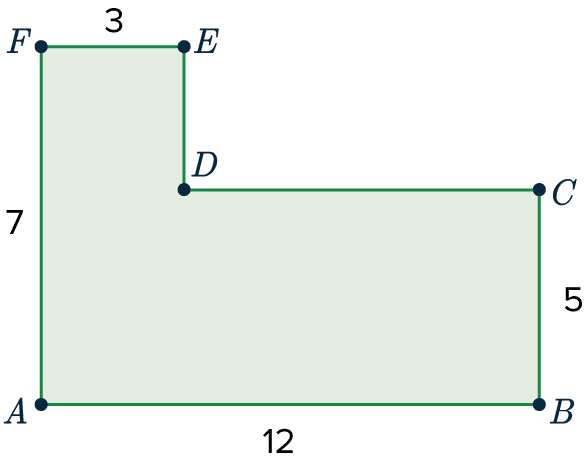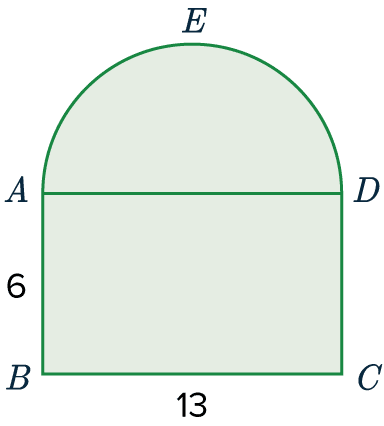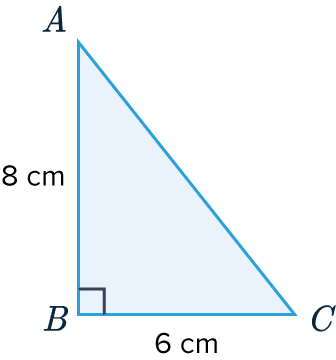# Perimeter

GCSEKS3Level 1-3Level 4-5AQACambridge iGCSEEdexcelEdexcel iGCSEOCRWJEC

## Perimeter

The perimeter of a 2D shape is the distance around the edges of the shape. It is found by adding up the lengths of each individual edge of the shape. This can be easy for simple shapes and harder for composite shapes.

Make sure you are happy with the following the following topics:

Level 4-5GCSEKS3AQAEdexcelOCRWJECCambridge iGCSEEdexcel iGCSE

## Perimeter of Simple Shapes

The rectangle shown below has a length of $11$cm and a width of $3$cm.

Calculate the perimeter of the rectangle shown.The opposite sides of a rectangle are equal in length.

So, the two sides that are not labelled must also be $3$ cm and $11$ cm.

Therefore, we get

Perimeter $= 3+3+11+11=28$ cm

Level 1-3GCSEKS3AQAEdexcelOCRWJECCambridge iGCSEEdexcel iGCSE

## Perimeter of Compound Shapes

$ABCDEF$ is a composite shape made up of rectangles.

Calculate the perimeter of shape $ABCDEF$.Usually, with compound shapes there will be some missing sides which need to be calculated.

First we need to calculate $ED$.

We can see that $FA = ED + CB$, so we can calculate:

$7 = 5 + ED$

$ED = 2$

Next, we do the same with $DC$.

$AB = FE + DC$

$12 = 3+DC$

$DC = 9$

Finally, we can calculate the perimeter by adding up all the side lengths.

$3 + 2+ 9 + 5+ 12+ 7 = 38$

Level 4-5GCSEKS3AQAEdexcelOCRWJECCambridge iGCSEEdexcel iGCSE

## Example 1: Compound Shapes

$ABCDE$ is a shape made up of a rectangle and a semi-circle.
$AB = 6,\,BC=13$.

Calculate the perimeter of this shape to $3$ significant figures.

[2 marks]With compound shapes we first need to break it up into two parts.

1) Rectangle – We know that rectangles have two pairs of equal sides, so we know that $DC=6$ and $AD=13$. However, since it is not on the outside of the shape, we won’t be counting $AD$ with the perimeter. Therefore, the perimeter so far (sides $AB$, $BC$, and $CD$) is

$6+13+6=25$

2) Semi-circle – The formula for the circumference of a circle is $\pi d$, where $d$ is the diameter. Because it is a semi-circle we’re dealing with, we will half the result. Doing this, we get

$AED=\dfrac{(\pi \times 13)}{2}=20.420...$

Now, adding the values together we get the total perimeter to be

$25+20.420...=45.4$ ($3$ sf)

Level 4-5GCSEKS3AQAEdexcelOCRWJECCambridge iGCSEEdexcel iGCSE

## Example 2: Perimeter of Triangles

$ABC$ is a triangle. $AB = 8$ cm, $BC = 6$ cm. Angle $ABC$ is a right-angle. Calculate the perimeter of triangle $ABC$.

[2 marks]

In this case, we are given two sides of the triangle but will have to work out the third if we want to find the perimeter. Since this is a right-angled triangle, we can use Pythagoras! Pythagoras’ theorem says

$a^2+b^2=c^2$,

Where $c$ is the hypotenuse and $a$ and $b$ are the other two sides. So, the equation becomes

$8^2+6^2=AC^2$

Evaluating the left-hand side, and then square rooting, we get

$AC^2=64+36=100$

$AC=\sqrt{100}=10\text{ cm}$

Now we have all three sides, simply add them to get the perimeter:

$6+8+10=24\text{ cm}$Level 4-5GCSEKS3AQAEdexcelOCRWJECCambridge iGCSEEdexcel iGCSE

## Perimeter Example Questions

To find the perimeter, we need to find the length of one side (all sides are the same, since it’s a square). If we say that $x$ is the length of one side, then the area is,

$x^2=64$

So, if we square root both sides, we find that $x=8$. Therefore, the perimeter of the square is,

$8+8+8+8=32\text{ m}$Gold Standard Education

This hexagon is regular, so all 6 of its sides must be the same length. Since the perimeter is the result of adding all the sides together, we get:

$\text{Length of one side}=21 \div 6=3.5\text{ cm}$Gold Standard Education

Perimeter of semi-circle arc can be calculated by finding half of the circumference of a complete circle:

$\dfrac{1}{2}\times\pi\times10=5\pi$ cm

Length of base $= 10$ cm

Total Perimeter $= 10 +5\pi = 25.7$cmGold Standard Education

To find the perimeter, we first need to find the missing lengths:

$120-55=65$ cm

$195-70=125$ cm

Hence adding all the lengths together:

Total Perimeter $=120+70+65+125+55+195=630$ cmGold Standard Education

Here we are given the perimeter, whilst the side-lengths are expressed in terms of $x$. Since this triangle is an isosceles triangle, we know that,

$AB = BC = x+5$

$(x+5) + (x+5) + 3x= 45 \text{cm}$

Now, to find $x$ we must solve this equation,

\begin{aligned}5x+10&=45 \\ 5x&=35 \\ x&= 35\div5=7\text{cm}\end{aligned}Gold Standard Education

## Perimeter Worksheet and Example Questions

### (NEW) Perimeter Exam Style Questions - MME

Level 4-5GCSENewOfficial MMEGold Standard Education

Level 1-3GCSE

Level 4-5GCSE